Crest Factor Role in Improving Power Quality

# Crest Factor Role in Improving Power Quality

## The Crest factor: The Purity of the Output Wave of the Network

Crest factor is one of the terms that is widely used in measuring the quality of power networks. It is a parameter that can be used to check the purity of the output wave of the network. Due to the presence of non-linear loads, devices, and industrial equipment in electrical networks, the harmonic injected by these components into the network is very high. Harmonics can have an inappropriate effect on the network, and for this reason, different parameters should be used to measure the quality of the network, of which the crest factor is one. In this article, we will learn the crest factor, its application, and its calculation method.

## What Is Crest Factor?

The mathematical definition of the crest factor is that it shows the ratio of the peak value of the waveform to its effective value. This definition is only a mathematical definition or calculation formula and does not include many components related to this parameter. In some cases, this parameter can also be defined as the peak-to-average ratio. This definition tells us how far the peak of the waveform is from its average value.

For a more technical and specialized definition of this parameter, we must say it can only be used for stable signals such as sine, square, saw, or triangle waves. It can determine the purity of a signal or waveform and the ability of systems such as power supplies to output a certain current or voltage.

The crest factor displays large peaks in a waveform. For example, the value of this parameter for weak DC systems with resistive load should be 1:1, which is the minimum value of the parameter. On the other hand, the value of this parameter for an AC sinusoidal waveform with a resistive load is 1.414. Some information technology equipment and other loads with modified power factor sources have a crest factor of about 1.414.

Meanwhile, unmodified equipment such as stackable hubs and personal computers have a factor of 2 or 3. For applications that require a pure sine wave, the power supply should have a crest factor of 1.414 or as close as possible. Distortion caused by interactions between supply and load may affect the relationship between the peak and RMS values and lead to a higher value of the parameter.

## Why Is the Crest Factor Important?

The crest factor for the current generated by non-linear loads is usually greater than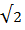. But it should be said that this parameter is generally between 1.5 and 2 and even in critical cases it can reach 5.

Considering that this parameter is a ratio of power, it is generally expressed in decibels. Now that we are familiar with the concept of this parameter, it is better to know a little about the importance of its calculation. In UPS systems, calculating this parameter is very important. Because the output voltage in these systems is generally created by the switching of semiconductor parts and they are highly sensitive to the passing current.

In this situation, if the load current drawn from the UPS systems is, for example, one amp, the crest factor of the load current may be six amps, and as a result, the ammeter shows the number one for both modes. But it should be said that the peak of the current passing through the output semiconductor parts of the system will be three in one order and will be six in another order.

Now, if the current peak is more than the tolerance range of the parts, these parts will burn and be destroyed in a very short time. Therefore, knowing this parameter in UPS systems and other components of the power grid is very important.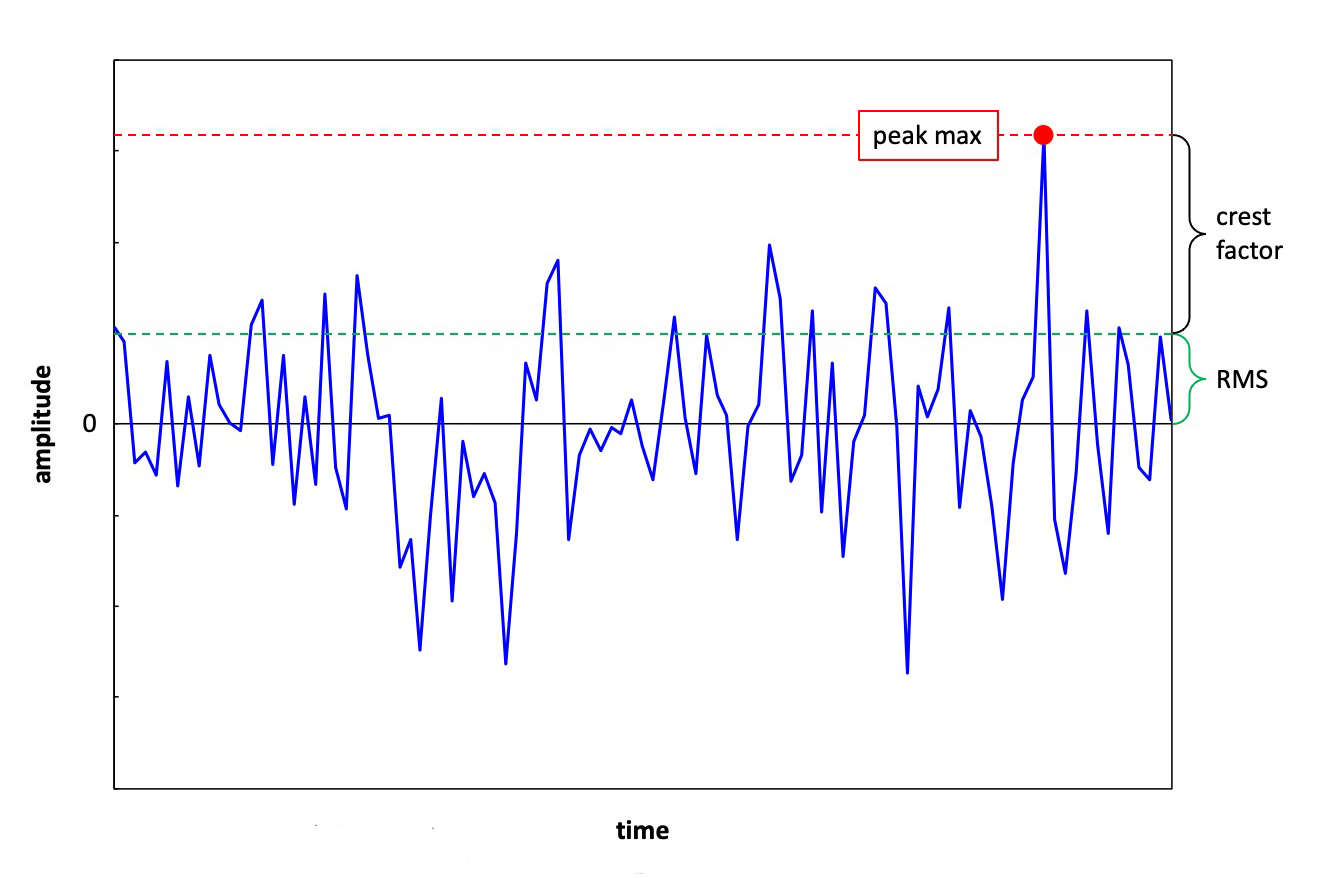The crest factor of a source indicates the probable and safe peak output currents it can handle above the rated current. Since the supplies can provide higher outputs, they must be equipped with safety circuits that turn off and then disconnect the power distribution if the load draws a high current.

Crest Factor Voltage (CF V)

Power generators provide a perfectly sinusoidal voltage waveform, but this waveform is usually not ideal at the moment it reaches the end user.

Clipping, sometimes referred to as "Flat-Topping", is a persistent problem in AC systems and occurs mostly in end-user installations behind the breaker panel when the wire resistance is too high, resulting in It is due to the length of the wire and inappropriate section and the use of non-linear loads.

A typical example is a switching power supply without power factor compensation (PFC). Here only the peak voltage is shaved or cut and we have a lower peak value. This results in a crest factor of less than 1/414.

Monitoring the voltage crest factor is an excellent approach to finding potential electrical problems in installations. For example, if the voltage crest factor of an electrical circuit is close to 1/4 when the circuit is unloaded, but drops to less than 1/1 when an electronic load is connected, this could be an indication that the wiring of the circuit is at the threshold or above the maximum power transmission capacity. Installing a new wire with a wider cross section will be a good solution in this situation.

Crest factor measurement can detect potential problems with incorrect cable and load size selection, while conventional voltage measurement cannot. For example, if you measure the voltage value of 227 V on a power line as 230 V, it looks good. But if at the same time the crest factor of the voltage is less than 1/4, it can be concluded that there is a circuit that is not serviced or overloaded, which can seriously damage the connected equipment, because it is not receiving the maximum power.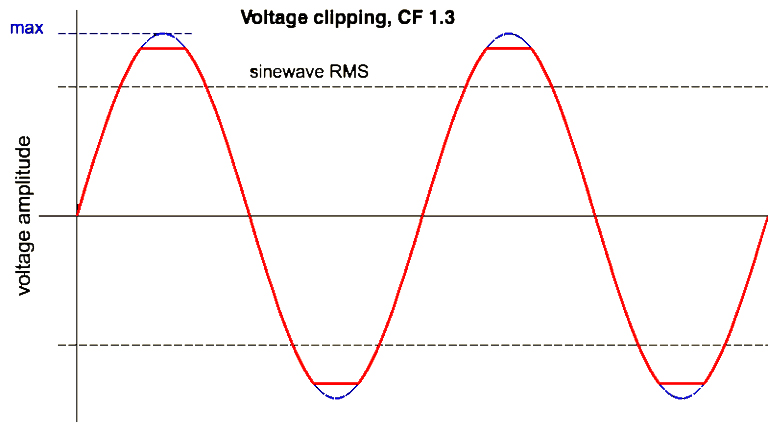Crest Current Factor (CF I)

The voltage waveform is generated as a sine wave, while the current drawn by electronic loads may not be sinusoidal. (Only pure resistive loads draw current in a sinusoidal pattern (in blue, CF I = 1/414).)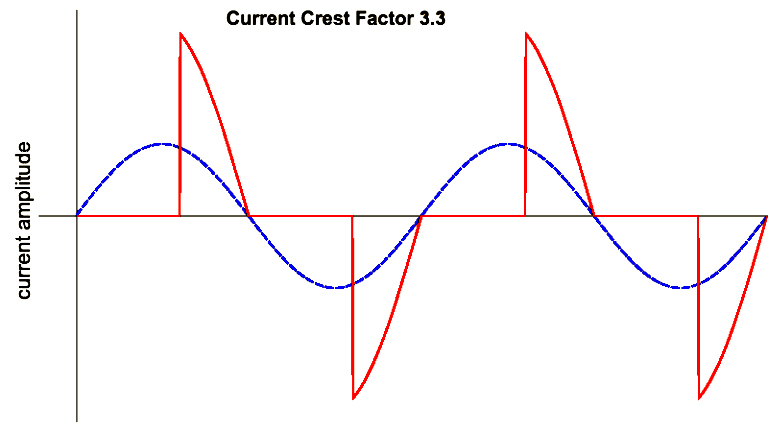For example, switching power supplies draw current at peak (red line). And uncompensated electronic loads can easily reach a CF greater than 3 as well.

## How Is the Crest Factor Calculated?

Before going to the calculation method, it is necessary to mention that the power supply must be able to supply the maximum current of the load. Otherwise, the source voltage will be changed by the excessive peak current. Most power supply manufacturers usually provide a crest factor to help consumers or designers match their loads to the right supplies.

As mentioned in the mathematical definition of the crest factor, to calculate this parameter, it is enough to divide the peak of the waveform by its effective value. In the explanation of the formula of this parameter, it should be mentioned that the peak is also known as peak value, maximum current, or peak and maximum value. Also, RMS is the oscillation amplitude of a sine wave. The crest factor value of DC voltages is 1 and in the triangular waveform, this value is equal to 1.73. Square waves also have a crest factor equal to 1. Because these waves have no peaks. In the figure below, you can see the exact formula for calculating this quality control parameter of the power grid.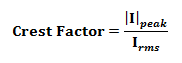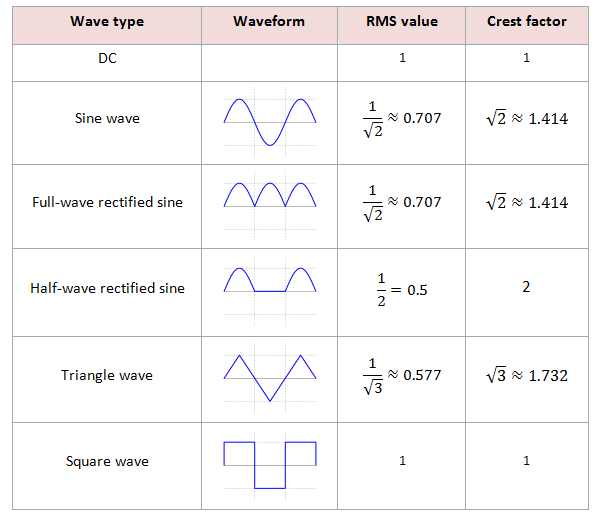Share